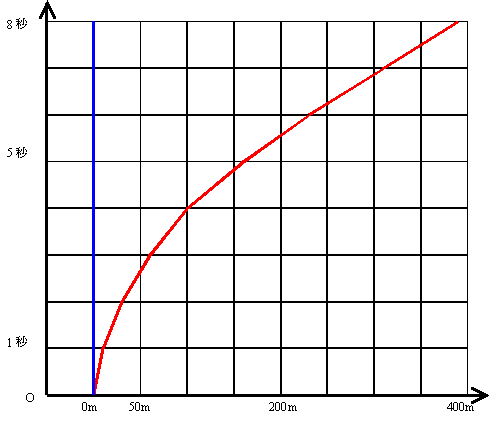# The equivalence principle and the cause of gravity

2014/2/11
Published 2013/2/7

The principle of equivalence is the idea that we cannot distinguish gravity from an inertial force due to the acceleration.
I would like to consider the cause of gravity in this page.## The equivalence principle

Einstein has had an inspiration of the equivalence principle which was the best idea of his lifetime in the patent office in 1907. The equivalence principle is the principle which is that the following two states are equivalent locally.

• State of the elevator accelerating upward
• State of being attracted to the Earth's gravity

For example, place the box of the elevator on the ground. Then we put the person in the box of the elevator. Finally, we inform to the person that the box of the elevator is floating in space.

The person is pressed against the floor of the elevator. For this reason, the person must infer that the box of the elevator is accelerating upward.

In other words, according to the equivalence principle, we are able to interpret that the space around us are falling toward the earth at the gravitational acceleration 9.8 [m/s2].

## What is the mechanism that gravity occurs?

I would like to consider the mechanism that gravity occurs in the perspective of the equivalence principle. We express the gravitational acceleration by Newton's gravitational equation as follows.

g=GM/R2

Here,g, G, M, and R are shown below.

 Gravitational acceleration g 9.8 [m/s2] Gravitational constant G 6.7×10-11 [m3/s2 kg] The mass of the Earth M 6.0×1024 [kg] The radius of the Earth R R 6400 [km]

We get the following equation by multiplying 4πR2 to both sides

4πR2g = 4πGM

In the perspective of the equivalence principle, we are able to interpret the left-hand side as that the earth is ingesting the volume 4πR2×9.8 [m3] at an accelerated rate on the spherical shell of radius R. On the other hand, we are able to interpret the right-hand side as that the earth is ingesting the volume 4π×6.7×10-11×M [m3] on the spherical shell of radius R at an accelerated rate.

Since our space is three-dimensional, accelerated ingestion attenuates in the proportion to the inverse-square of the distance.

## Space-time distortion by gravity

Einstein went on thinking eight years a mathematical representation of this equivalence principle. And Einstein saw through that he expresses it by bending of space-time, space-time distortion at last. I think Einstein is genius. Thus he completed the general theory of relativity in 1915.

I will explain the state of the space-time distortion in the following figure.

The blue line represents the object that is stationary in the space of zero gravity. The red line represents the object that is falling on the right side in the gravitational field.If we interpret a moving object is stationary in the space of zero gravity, we are able to interpreted the trajectory of a moving object as the bending of space-time.

In order to explain the general theory of relativity, a distorted sheet that represents a space is often used. However, actually not space, but space-time is distorted.

## The general theory of relativity

In order to explore the relationship of a material and distorted space-time, I would like to look at the gravity equation of Einstein as shown below.

Gμν=8πGTμν

Gμν on the left-hand side is a geometric quantity. Tμν on the right-hand side is a material quantity. From the equation, we see that the material is bending the space-time. Here, I would like to quote from the book "Physics and reality" written by Einstein in 1936.

[General Relativity] is suﬃcient — as far as we know — for the representation of experiences of celestial mechanics. But it is similar to a building, one wing of which is made of ﬁne marble (left side of the equation), but the other wing of which is built of low-grade wood (right side of equation). The phenomenological representation of matter is, in fact, only a crude substitute for a representation which would do justice to all known properties of matter.

I think the representations "one wing of which is made of ﬁne marble" and "the other wing of which is built of low-grade wood" are symbolic. Maybe we should write the right-hand side by a geometric quantity as well. I think we should express the material by geometric principles.

## Why does gravity occur?

What is the cause of gravity? Gravity equation of Einstein tells us the relationship of space-time distortion and materials. However, it does not tell us the cause of material to bend time and space. How do materials bend the space-time?

Mathematician Clifford said the following in a paper "On the Space-Theory of Matter" to the Cambridge Philosophical Association in 1870.

1. That small portions of space are in fact of a nature analogous to little hills on a surface which is on the average flat; namely, that the ordinary laws of geometry are not valid in them.
2. That this property of being curved or distorted is continually being passed on from one portion of space to another after the manner of a wave.
3. That this variation of the curvature of space is what really happens in that phenomenon which we call the motion of matter, whether ponderable or ethereal.

In other words, materials might be a distorted space. If materials are a distorted space, we might be able to explain the cause of the gravity because the both left-hand side right-hand side are distorted space. As a final conclusion, the cause of gravity is a mystery. How would you like to try to challenge this mystery?

Related Articles: